# Container

How many three-quarters liters jar fill 30 liters container?

n =  40

### Step-by-step explanation:

$n=30\mathrm{/}\left(3\mathrm{/}4\right)=40$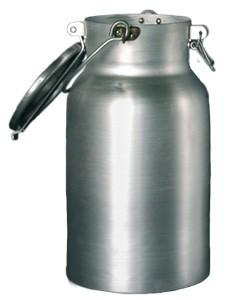Did you find an error or inaccuracy? Feel free to write us. Thank you!Tips to related online calculators
Need help to calculate sum, simplify or multiply fractions? Try our fraction calculator.
Tip: Our volume units converter will help you with the conversion of volume units.

## Related math problems and questions:

• WillieWillie collected 6 2/3 pails of water. How many big containers can she fill? Does each container contain 2 2/9 of a pail?
• Motor oil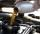30 liters of oil cost 28.80 Euros. How much cost a liter?
• Liters in cylinderDetermine the height at which level 24 liters of water in a cylindrical container having a bottom diameter 36 cm.
• What fraction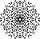What fraction of number 1 to 30 is prime?
• Paula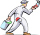Paula is painting six doors that are all the same size. She used 2 liters of paint to cover 1 3/5 doors. How many liters of paint are needed for 1 door?
• AquariumHow many liters of water can fit in an aquarium measuring 30, 15 and 20 cm?
• Pumps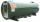6 pump fills the tank for 3 and a half days. How long will fill the tank 7 equally powerful pumps?
• Cuboid aquariumCuboid 25 times 30 cm. How long is third side if cuboid contains 30 liters of water?
• AquariumCan 30 liters of water fit in a cuboid aquarium with dimensions a = 3dm b = 6dm c = 5dm?
• How manyHow many integers are greater than 547/3 and less than 931/4?
• Oil change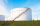A container holds 1/4 gallon of oil. Jack is changing the oil in his vehicles and needs 11 containers. How many gallons of oil does Jack need?
• A glass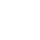A glass holds 3/4 cups. A jug holds 12 cups. How many glasses can be filled?
• How many 16How many three-tenths are there in two and one-fourths?
• Sort fractions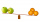Which of the following fractions is the largest? 29/36 5/6 7/9 3/4
• Water overflow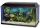A rectangular container that has a length of 30 cm, a width of 20 cm, and a height of 24 cm is filled with water to a depth of 15 cm. When an additional 6.5 liters of water are poured into the container, some water overflows. How many liters of water over
• Container with water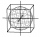The cube-shaped container is filled with water to half its height. If we add 20 liters of water, the container will be filled to three-quarters of its height. What is the volume of the whole container?
• Painting classroomsMartha solicited 3 2/3 liters of paint for the brigade. Their city mayor gave their school another 2 2/5 liters of paint. If each classroom needs 1 3/7 liters, how many classrooms can be painted?